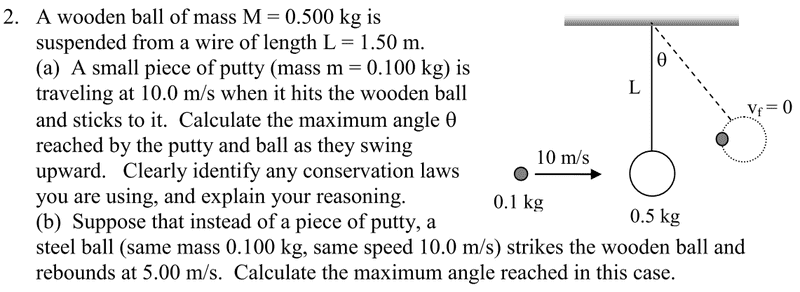# Conservation Laws - Finding angle ?

• nukeman
In summary, to find the angle in question 2a, you can use the conservation laws of momentum and energy. First, use conservation of momentum to find the velocity immediately after the putty sticks to the wood. This velocity will give you the kinetic energy of the wood and putty combined. Next, use conservation of energy (potential energy vs kinetic energy) to find the height that the wood and putty can rise to. Finally, use geometry to find the angle.

#### nukeman

Conservation Laws - Finding angle ?

## Homework Statement

Having trouble with question 2, a) - See below## The Attempt at a Solution

Which conservations laws am I suppoe to use to figure this out?

THanks!

nukeman said:
Which conservations laws am I suppoe to use to figure this out?

What conservation laws do you know? Which ones are usually applied to kinematic problems?

EDIT: @ehild: Spooky.

Last edited:

You can use the conservation of momentum law that states the momentum before the collision is equal to the momentum after the collision. momentum being equal to mass*velocity.

You can use the law that states that potential energy before the action is equal to the kinetic energy after the action to find the height in which the ball traveled upward.

Those were the only two that I needed to solve the problem, good luck!

Potential, can you tell me the formula you used?

Im still having trouble with A), finding the angle?

Use conservation of momentum to find the velocity immediately after the putty sticks to the wood.

That velocity gives you the KE of the wood+putty. After that, conservation of energy (Gravitational PE vs KE) will tell you how high the wood+putty can rise. After that it's just geometry.

Why don't you start by writing the expression for conservation of momentum that pertains to the collision?

I used the formula PE=mgh and KE=(mv^2)/2
the law states that the potential energy at the top is equal to the kinetic energy at the bottom, so mgh=(mv^2)/2

and as for the law of momentum, Pbefore = Pafter (p is momentum) so before the collision, the only thing moving was the putty. after the collision, both the putty and the wooden ball are moving. so, Mputty*Vputty=(Mputty+Mball)*V (the velocity would be the new velocity of the ball and putty combined)